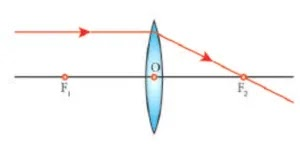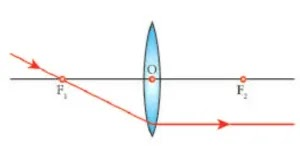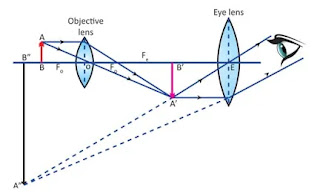# 10th Science Book Back Answer Unit 2 OPTICS

## 10th Standard Science Physics Lesson 2 OPTICS Book Back Answers | Samacheer Kalvi Guide

10th Science Book Back Answer Unit 2. OPTICS. 10th Standard Science Physics Answers, 10th Chemistry Book Back Answers, 10th Biology Book Back Answers Tamil Medium and English Medium. 10th All Subject Text Books. Class 10 Science Samacheer kalvi guide. 10th Tamil Samacheer Kalvi Guide. 10th Science Unit 2. OPTICS Book back answers.## 10th Standard Science Book Back Answers Lesson 2 OPTICS

 Formulae Velocity of light C = vλ Rayleigh’s Scattering Law i S α 1/λ4 Lens formula 1/f = 1/v-1/u Magnification m = h/h = v/u = distance of the image / distance of the objec Power of lens p= 1/f (f in meter) Len’s makers formula 1/f=(μ – 1)(1/R1 – 1/R2) Focal length of required concave lens for myopia f = xy / x – y Focal length of the required convex lens for hypermetropia f = dD / d – D Visual angle, (tan θ) height/distance Angular magnification M = α / β α – angle subtended by the final image at the eye. β – angle substended by the object at the naked eye In simple microscope M = 1 + D/f D → Least distance of distinct vision from the lens of eye Compound microscope magnification m = (v/fo-1) (1+D/fe) M=Mo x Me Astronomical telescope Magnification M = fo/fe

## TEXTBOOK EVALUATION

### I. Choose the correct answer

1. a) A
2. b)B
3. c) C
4. d) D

Ans ; A

#### 2. Where should an object be placed so that a real and inverted image of same size is obtained by a convex lens

1. f
2. 2f
3. infinity
4. between f and 2f

Ans ; 2f

#### 3. A small bulb is placed at the principal focus of a convex lens. When the bulb is switched on, the lens will produce

1. a convergent beam of light
2. a divergent beam of light
3. a parallel beam of light
4. a coloured beam of light

Ans ; a parallel beam of light

#### 4. Magnification of a convex lens is

1. Positive
2. negative
3. either positive or negative
4. zero

Ans ; either positive or negative

#### 5. A convex lens forms a real, diminished point sized image at focus. Then the position of the object is at

1. focus
2. infinity
3. at 2f
4. between f and 2f

Ans ; infinity

1. 4m
2. –40m
3. –0.25 m
4. –2.5 m

Ans ; –0.25 m

#### 7. In a myopic eye, the image of the object is formed

1. behind the retina
2. on the retina
3. in front of the retina
4. on the blind spot

Ans ; in front of the retina

#### 8. The eye defect ‘presbyopia’ can be corrected by

1. convex lens
2. concave lens
3. convex mirror
4. Bifocal lenses

Ans ; Bifocal lenses

#### 9. Which of the following lens would you prefer to use while reading small letters found in a dictionary?

1. A convex lens of focal length 5 cm
2. A concave lens of focal length 5 cm
3. A convex lens of focal length 10 cm
4. A concave lens of focal length 10 cm

Ans ; A convex lens of focal length 5 cm

#### 10. If VB, VG, VR be the velocity of blue, green and red light respectively in a glass prism, then which of the following statement gives the correct relation?

1. VB = VG = VR
2. VB > VG >VR
3. VB < VG < VR
4. VB < VG > VR

Ans ; VB > VG >VR

### III. True or False. If false correct it.

1. Velocity of light is greater in denser medium than in rarer medium ( False )
• Correct Statement: Velocity of light is lseser in denser medium than in rarer medium.
1. The power of lens depends on the focal length of the lens ( True )
2. Increase in the converging power of eye lens cause ‘hypermetropia’ ( True )
3. The convex lens always gives small virtual image. ( False )
• Correct Statement : The concave lens always gives small virtual image
1. Match the following:

Column – I –   Column – II

1) Retina    –    a) Path way of light

2) Pupil        –  b) Far point comes closer

3) Ciliary muscles       –    c) near point moves away

4) Myopia     –      d) Screen of the eye

5) Hypermetropia   –   f) Power of accommodation

Ans ; 1 – d, 2 – a, 3 – e, 4 – b, 5 – c

### V. Assertion and reasoning type Mark the correct choice as

1. If both assertion and reason are true and reason is the correct explanation of assertion.
2. If both assertion and reason are true but reason is not the correct explanation of assertion.
3. Assertion is true but reason is false.
4. Assertion is false but reason is true.

1. Assertion: If the refractive index of the medium is high (denser medium) the velocity of the light in that medium will be small

Reason: Refractive index of the medium is inversely proportional to the velocity of the light

Ans : If both assertion and reason are true and reason is the correct explanation of assertion.

1. Assertion: Myopia is due to the increase in the converging power of eye lens.

Reason: Myopia can be corrected with the help of concave lens.

Ans : If both assertion and reason are true and reason is the correct explanation of assertion.

#### 1, What is refractive index?

The ratio of speed of light in vacuum to the speed of light in a medium is defined as refractive index (μ) of the medium.

μ = sin i / sin r

#### 2. State Snell’s law.

The ratio of the sine of the angle of incidence and sine of the angle of refraction is equal to the ratio of refractive indices of the two media. This law is also known as Snell’s law.

sin i/sin r = μ2/μ1

#### 3. Draw a ray diagram to show the image formed by a convex lens when the object is placed between F and 2F.#### 4. Define dispersion of light.

When a beam of white light or composite light is refracted through any transparent media such as glass or water it is split into its component colours. This phenomenon is called as dispersion of light.

#### 5. State Rayleigh’s law of scattering.

Rayleigh’s scattering law states that “The amount of scattering of light is inversely proportional to the fourth power of its wavelength.

Amount of scattering = ‘s’ α 1/λ4

#### 6. Differentiate convex lens.and concave lens.

 Convex lens Concave lens A convex lens is thicker in the middle than at edges. A concave lens is thinner in the middle than at edges. 2. | Itis a converging lens It is a diverging lens. 3. It produces mostly real images. It produces virtual images. 4. It is used to treat hypermetropia It is used to treat myopia.

#### 7. What is power of accommodation of eye?

The ability of the eye lens to focus nearby as well as the distant objects is called power of accommodation of the eye.

#### 8. What are the causes of ‘Myopia’?

• The focal length of eye lens is reduced or the distance between eye lens and retina increases.
• Hence the far point will not be infinity for such eyes and the far point has come closer, with this defect, nearby object can be seen clearly but distant objects cannot be seen clearly.

#### 9. Why does the sky appear in blue colour?

• When sunlight passes through the atmosphere the blue colour (Shorter wavelength) is scattered to a greater extent than the red colour (larger wavelength).
• This scattering causes the sky to appear in blue colour.

#### 10. Why are traffic signals red in colour?

• The red colour has longer wavelength.
• So it can travel for longer distance and will be seen clearly. \$o red colour is used in traffic signals.

### VII. Give the answer in detail:

#### 1. List any five properties of light

Properties of light:

• Light is a form of energy.
• Light always travels along a straight line.
• Light does not need any medium for its propagation. It can even travel through vacuum.
• The speed of light in vacuum or air is, c = 3 x 108 m/s’
• Since, light is in the form of waves, it is characterized by a wavelength (λ) and a frequency (v), which are related by the following equation: c = vλ (c – velocity of light).

#### 2. Explain the rules for obtaining images formed by a convex lens with the help of ray diagram.

Rules for obtaining images:

• When an object is placed infront of a lens, the light rays from the object fall on the lens.
• The position, size and nature of the image formed can be understood only if we know certain basic rules.

Rule-1:

When a ray of light strikes the convex lens obliquely at its optical centre, it continues to follow its path without any deviation.Rays passing through the optical centre

Rule-2:

When rays parallel to the principal axis strikes a convex lens, the refracted rays are converged to (convex lens) the principal focus.Rays passing parallel to the optic axis

Rule-3:

When a ray passing through (convex lens) the principal focus strikes a convex or concave lens, the refracted ray will be parallel to the principal axisRays passing through or directed towards the principal focus

#### 3. Differentiate the eye defects: Myopia and Hypermetropia.

 Myopia Hypermetropia 1. Short sightedness Long sightedness 2. Occurs due to lengthening of eye ball Occurs due to shortening of eye ball. 3. Nearby objects can be seen clearly Nearby objects cannot be seen clearly 4. Distant objects cannot be seen clearly Distant objects can be seen clearly 5. The focal length of eye lens is reduced The focal length of eye lens is increased 6. The far points will not be infinityfor such eyes and the far points have come closer The near points will not be at 25 cm for such eyes and the near point have moved farther 7. The image of distant objects are formed before the retina The image of nearby objects are formed behind the retina 8. The defect can be corrected using concave lens of negative power The defect can be corrected using convex lens of positive power

#### 4, Explain the construction and working of a ‘Compound Microscope.

Compound microscope:

Construction:

• A compound microscope is used to see the tiny objects has better magnification power than simple microscope.
• A compound microscope consists of two convex lenses.
• The lens with the shorter focal length is placed near the object, and is called as ‘objective lens’ or ‘objective piece.
• The lens with larger focal length and larger aperture placed near the observer’s eye is called as ‘eye lens’ or ‘eye piece.
• Both the lenses are fixed in a narrow tube with adjustable provision.

Working:

• The object (AB) is placed at a distance slightly greater than the focal length of objective lens (u > fo).
• A real, inverted and magnified image (A’ B’) is formed at the other side of the objective lens.
• This image behaves as the object for the eye lens.
• The position of the eye lens is adjusted in such a way, that the image (A’ B’) falls within the principal focus of the eye piece.
• This eye piece forms a virtual, enlarged and erect image (A” B”) on the same side of the object.
• Compound microscope has 50 to 200 times more magnification power than simple microscope.Image formation in compound microscope

### VIII. Numerical Problems:

#### 1. An object is placed at a distance 20 cm from a convex lens of focal length 10cm. Find the image distance and nature of the image.

Solution:

Formula used:

Image distance 1/v = 1/f + 1/u

Given :

f= 10cm, u=-20cm, v=?

 1/f = 1/v – 1/u 1/v – 1/u = 1/f 1/v = 1/f + 1/u = 1/10 + 1/-20 = 1/10 – 1/20 1/v = 2-1/20 = 1/20 Image Distance v = 20 cm

Nature of image:

Real inverted is formed at the centre of curvature. The size of the image is same

#### 2. An object of height 3cm is placed at 10cm from a concave lens of focal length 15cm. Find the size of the image.

Solution:

Formula used:

Image distance 1/v = 1/f + 1/u

Given :

 1/f = 1/v – 1/u 1/v – 1/u = 1/f 1/v = 1/f + 1/u 1/v = 1/15 + 1/-10 = 1/15 + 1/10 1/v = -(2+3)/30 = -5/20 = -1/6 v = -6 cm

Size of the image

 Formula used : Magnificatio = +v/u Magnification = +v/u (m=h’/h) h’/h = -v/u h’/3 = -6/-10 = 0.6 Size of the image h’ = 0.6 x 3 = 1.8 cm

### IX. Higher Order Thinking Skills (HOTS)

#### 1. While doing an experiment for the determination of focal length of a convex lens, Raja Suddenly dropped the lens. It got broken into two halves along the axis. If he continues his experiment with the same lens, (a) can he get the image? (b) Is there any change in the focal length?

1. Yes he got the image. But the image is not clear.
2. No change in the focal length. Because no change in radius of curvature.

#### 2. The eyes of the nocturnal birds like owl are having a large cornea and a large pupil. How does it help them?

• The eyes of the nocturnal birds like owl have a large cornea and a large pupil. These features increase their field of vision and also shows an increase in retinal surface and help them to collect more ambient light during night.